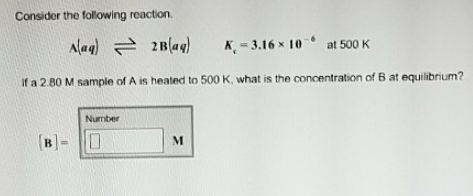# Problem: Consider the fllowing reaction. A(aq) ⇌ 2B(aq)             Kc = 3.16 x 10-6 at 500 KIf a 2.80 M sample of A is heated to 500 K, what is the concentration of B at equilibrium?

###### FREE Expert Solution
85% (173 ratings)###### Problem Details

Consider the fllowing reaction.

A(aq) ⇌ 2B(aq)             Kc = 3.16 x 10-6 at 500 K

If a 2.80 M sample of A is heated to 500 K, what is the concentration of B at equilibrium?